• ## PySpark教程

千次阅读 2018-12-20 09:40:13
PySpark简介 PySpark环境设置 PySpark SparkContext PySpark RDD PySpark广播与累积器 PySpark SparkConf PySpark SparkFiles PySpark StorageLevel PySpark MLlib PySpark Serializers


PySpark简介
PySpark环境设置
PySpark SparkContext
PySpark RDD
PySpark广播与累积器
PySpark SparkConf

PySpark SparkFiles
PySpark StorageLevel
PySpark MLlib
PySpark Serializers


展开全文• pyspark-tutorial：大学提供的pyspark教程的Jupyter笔记本
• PySpark简介 PySpark环境设置 PySpark SparkContext PySpark RDD PySpark广播与累积器 PySpark SparkConf PySpark SparkFiles PySpark StorageLevel PySpark MLlib PySpark Serializers
PySpark简介
PySpark环境设置
PySpark SparkContext
PySpark RDD
PySpark广播与累积器
PySpark SparkConf
PySpark SparkFiles
PySpark StorageLevel
PySpark MLlib
PySpark Serializers

展开全文python
• 用于大数据分析的PySpark备忘单 对于本文，我们使用了上公开可用的笔画预测数据集。 本教程包含以下主题： 加载数据中 查看资料 选择数据 计数数据 独特价值 筛选资料 订货数据 创建新变量 删除资料 更改数据类型 ...Python
• 本博客是【pySpark教程】系列的文章。 是 Berkeley 的 Python Spark公开课的学习笔记(see 原课程)。 由于个人能力有限，不免有些错误，还望各位批评指正。 更多相关博客请猛戳：...
Big Data, Hardware trends, and Spark

本博客是【pySpark教程】系列的文章。

是 Berkeley 的 Python Spark公开课的学习笔记(see 原课程)。

由于个人能力有限，不免有些错误，还望各位批评指正。

更多相关博客请猛戳：http://blog.csdn.net/cyh24/article/category/6092916

如需转载，请附上本文链接：http://blog.csdn.net/cyh_24/article/details/50659856

在本系列课程中，我们会学习如下内容：

Apache Spark 介绍 Big Data, Hardware trends, and Spark
Spark 要点
实验一：Spark 基础学习
Data Management Semi-Structed Data
Structured Data
实验二：使用 Spark 分析网络服务器日志
数据分析与机器学习 数据处理
数据分析
机器学习
实验三：文本分析与实体解析
实验四：Spark 机器学习介绍
The Big Data Problem

传统的数据分析的工具有下面这些，包括：Unix shell命令，Pandas 和 R 语言等等。这些工具都是运行到单个机器上的，遇到Big Data Problem 的时候就不太能work了。

那么 Big Data Problem 是啥呢？

数据增加速度大于计算性能
数据源越来越丰富
>> Web, mobile, scientific,…
存储变得越来越便宜
>> 基本上每18个月便宜一半
但是CPU性能的增长速度却达不到这样的水平
举一些 Big Data Examples可以看到，从disk中读取 1TB 数据需要3个小时，而且单个机器已经很难处理这样规模的数据了，一个解决方法就是把数据分布到大型集群中去。

Hardware for Big Data

如果集群使用的是廉价的机器，那么很容易发生一些问题：

1~5% 硬盘会损坏/年
0.2% 内存条损坏/年
Network 速度 VS 共享内存
从网络中读取的速度远远小于从硬盘或者内存中读取的速度
Uneven performance
机器的性能不均，有些机器很快，有些则计算的很慢
Distributing Work

集群的计算有没有困难的地方？

第一个challenge就是，如何将任务分配到不同的机器中？

来看一个例子（统计词频）：

1. 文件不是很大的情况下：
很简单，使用一个hash 表就能解决问题了。2. 文件很大的情况下：
这种情况下，其实也很简单，就是使用MapReduce 的思想，把数据map之后处理，然后再reduce结果。上图貌似可以解决问题了，但是，当数据特别大的时候，machine 5 的压力特别大，因为它要保存所有的结果（可能会存不下）。

这种情况下，可以采用下面这种分而治之的思想，把结果也分布到不同的机器上：这就是 Google 在04年提出的 Map Reduce：有便捷，肯定也会有缺陷，使用这种分而治之思想，会带来哪些问题呢？

数据的传输非常耗时
处理更多的机器意味着你需要解决更多的机器故障带来的问题
Solution：当一台机器故障的时候，你可以将这个未完成的任务分配给其他机器，或者等到这台机器恢复的时候再重新分配给它；
机器多了，性能差距也会变大，所以，你还需解决性能不均带来的问题
Solution：如果有一台机器非常慢，一直无法完成任务，那么你可以杀掉这个任务，并将它分配给其他机器；
所以，没有什么万能方法，你想要达到一些便利，就需要面对由此而来的困扰。

Map Reduce

Map Reduce 在每一次任务完成之后，都要把结果写入硬盘，并在下一次任务开始再读进来。如果我们的job是迭代式的（比如，机器学习中的迭代优化），那么计算性能就会非常慢。因为，每一次的迭代，你都需要重新读写。我们都知道，读写硬盘是一件非常非常耗时的事情。

Apache Spark

随着内存价格越来越低，我们可以更多的利用内存来进行计算。Spark 正是利用了内存速率高的特点，大大改进了Map Reduce的性能。

下图是 MapReduce 的过程：下图是 Spark 的过程：避免频繁的网络、硬盘读取，使得Spark速度大大提升。

Spark 发展到现在已经非常成熟，它提供了很多的数据分析工具，如下图：这些不同之处，带来了一些性能上的提升如下：但不同于MapReduce的是Job中间输出结果可以保存在内存中，从而不再需要读写HDFS。

Spark 能更好地适用于数据挖掘、机器学习等需要迭代优化的 MapReduce 的算法。

展开全文python spark
• 大数据架构基础知识 HDFS,yarn,mapreduce,spark,hive spark 1.简介 spark是一种计算引擎，类似于hadoop架构下mapreduce，与mapreduce不同的是将...spark是由scala语言开发，具备python的接口，pyspark。 2.spark组件
大数据生态圈简介
大数据生态圈可以分为7层，总的可以归纳为数据采集层、数据计算层和数据应用层。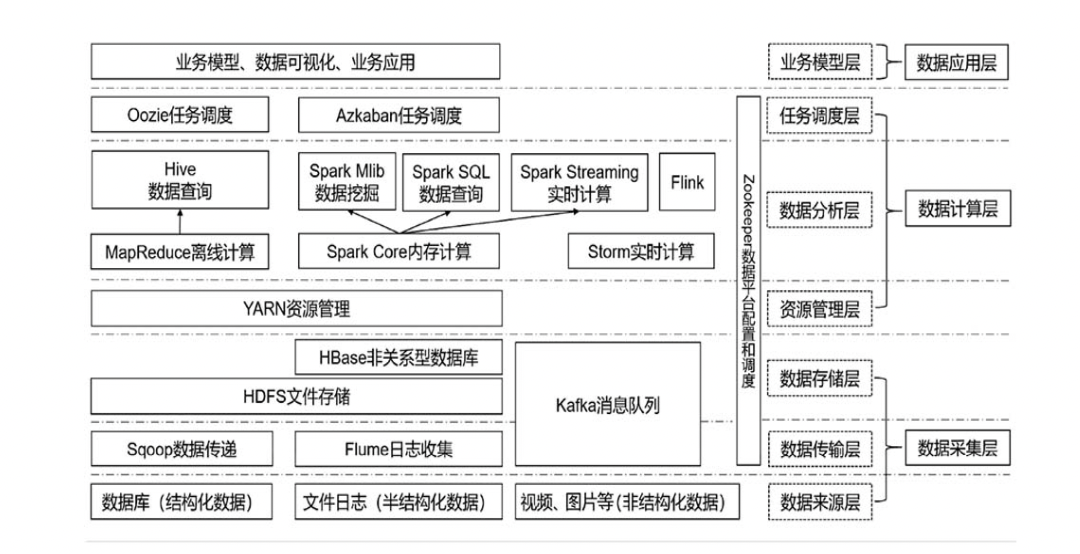spark
1.简介
spark不单单支持传统批量处理应用，更支持交互式查询、流式计算、机器学习、图计算等各种应用，
spark是由scala语言开发，具备python的接口，pyspark。
2.spark组件
spark包含着多个紧密集成的组件，如图所示：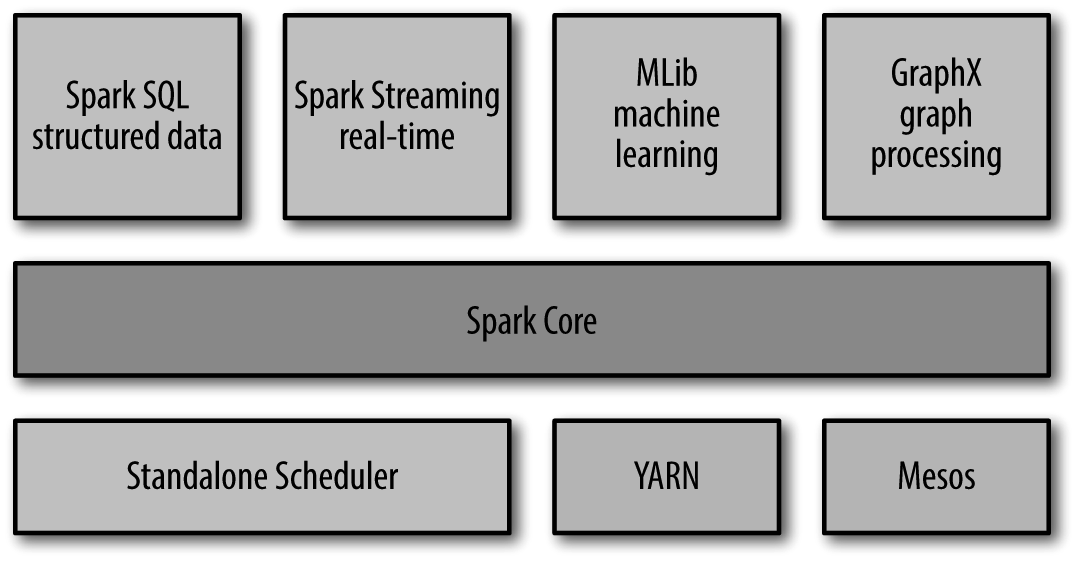2.1 spark core
实现spark基本功能，包含任务调度、内存管理、错误恢复、与存储系统交互等模块。
同时也包含对弹性分布式数据集（RDD），RDD表示分布在多个计算节点上可以并行操作的元素集合。
2.2 spark sql
spark sql用来操作结构化数据的程序包，我们可以使用sql或者hive语言来查询数据。
2.3 spark streaming
spark streaming上对实时数据进行流式计算的组件。例如：在网页服务日志，或者在网络服务中用户提交的状态更新组成的队列。
2.4 mlib
mlib提供机器学习功能程序库，提供多种机器学习算法
2.5 graphx
Graphx用来操作图，可以进行并行的图计算
2.6 集群管理器
Spark 设计为可以高效地在一个计算节点到数千个计算节点之间伸缩计
算。为了实现这样的要求，同时获得最大灵活性，Spark 支持在各种集群管理器。
搭建spark集群

步骤3：构造分布式spark集群

3.RDD编程
3.1RDD基础
实例1：读取外部数据集，并调用转化操作filter提取包含“python”的字符串，并调用first()行动，返回第一个包含python的字符串。
#初始化SparkContext
import pyspark
from pyspark import SparkContext,SparkConf

#配置应用
conf=SparkConf().setMaster("local").setAppName("My App")
#基于sparkconf创建一个sparkcontext
sc=SparkContext(conf=conf)
#读取外部数据
pythonlines=lines.filter(lambda line:"python" in line)
pythonlines.first()

out：u'## Interactive Python Shell'

实例2:spark的RDD会对每次行动进行重新计算，如果想复用同一个RDD，使用RDD.persist(),将RDD内容保存到内存中
pythonlines.persist
pythonlines.count()
pythonlines.first()

out:u'## Interactive Python Shell'

3.2创建RDD
实例1:将程序中一个已有集合传递给SparkContext的parallelize()
#内部创建数据
lines=sc.parallelize(["pandas","i like pandas"])
#外部读取数据

3.3RDD操作
3.3.1 转化操作
实例1:假定有一个日志文件log.txt，内部含若干信息，希望提取出其中的错误信息
inputRDD=sc.textFile("log.txt")
errorsRDD=inputRDD.filter(lambda x:"error" in x)

实例2:打印包含error或warning的行数
errorsRDD=inputRDD.filter(lambda x:"error" in x)
warningsRDD=inputRDD.filter(lamdba x:"warning" in x)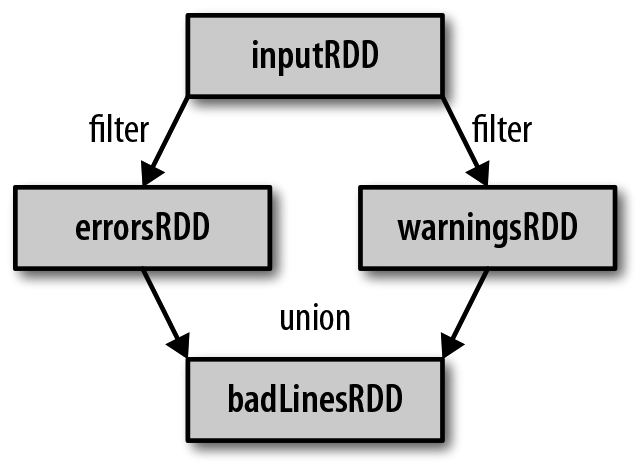3.3.2 行动操作
print("Input had"+badlinesRDD.count()+"concerning lines")
print("here are 10 examples:")
print line

3.4向spark传递函数
实例1:
#1
word=rdd.filter(lambda s:"error" in s)

#2
def containserrors(s):
return "error" in s
word=rdd.filter(containserror)

实例2:
class wordfunctions(object):
def getmatchesnoreference(self,rdd):
query=self.query
return rdd.filter(lambda x:query in x)

3.5常见转化操作和行动操作
3.5.1 基本RDD
map()和filter()
实例1:计算RDD中各值的平方
nums=sc.parallelize([1,2,3,4])
squared=nums.map(lambda x:x*x).collect()
for num in squared:
print "%i "(num)

实例2：使用flatMap()将行数据划分为单词
lines=sc.parallelize(["hello world","hi"])
words=lines.flatMap(lambda line:line.split(" "))
words.first()

其他转化操作：
集合操作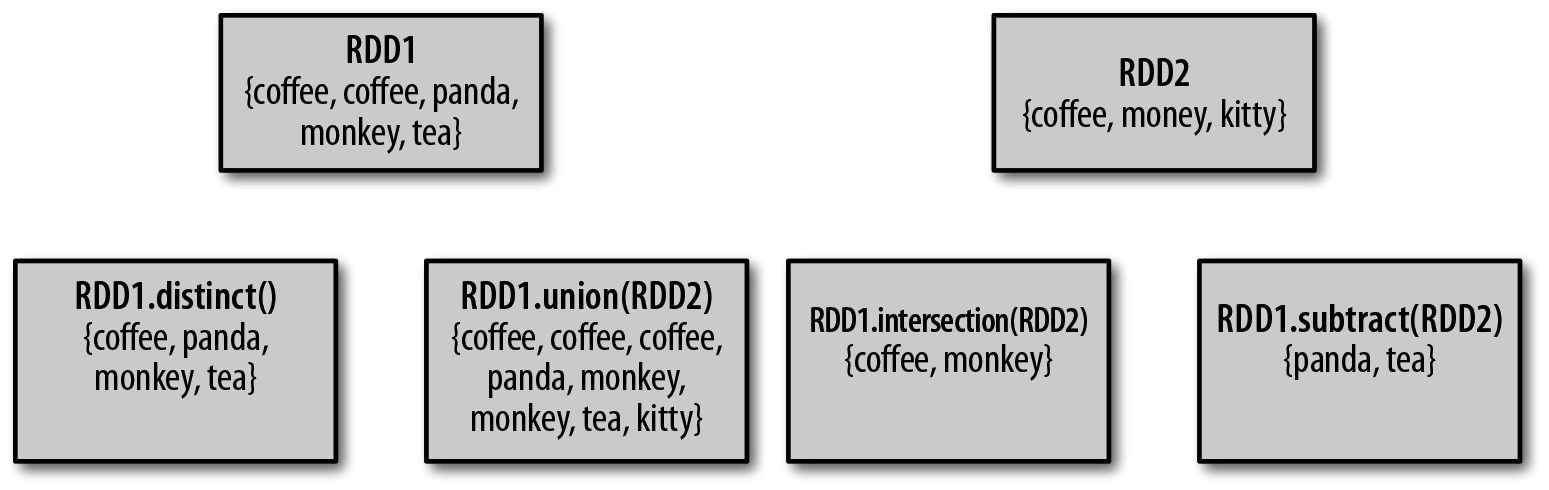RDD笛卡儿积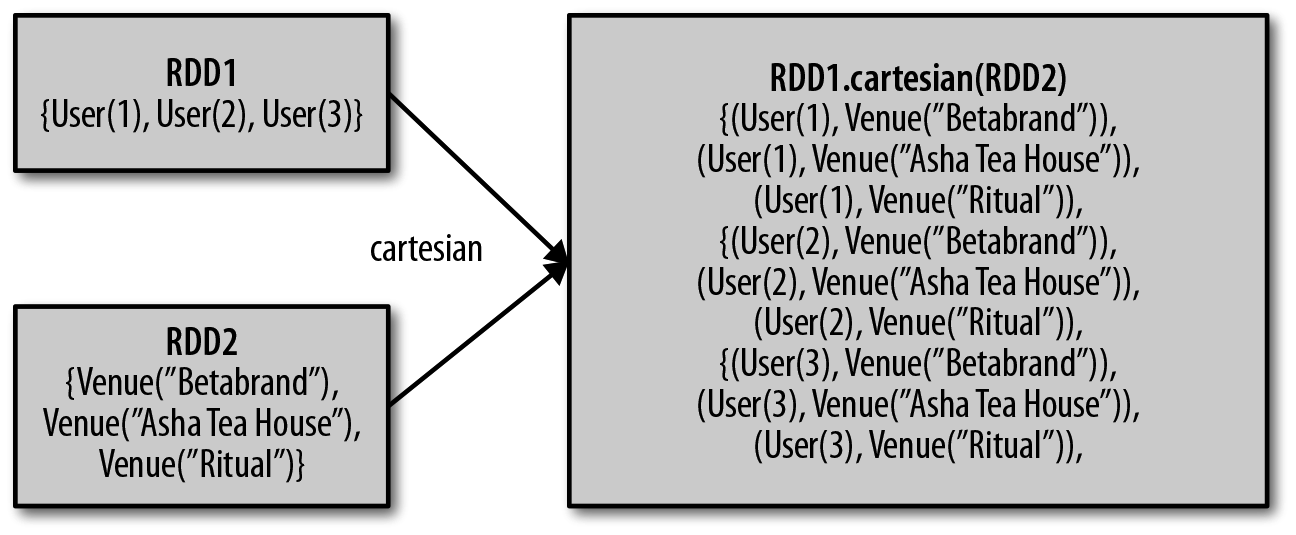转化操作列表

函数名
目的
示例
结果

map()
将函数应用于RDD 中的每个元素，将返回值构成新的RDD
rdd.map(x => x + 1)
{2, 3, 4, 4}

flatMap()
将函数应用于RDD 中的每个元素，将返回的迭代器的所有内容构成新的RDD。通常用来切分单词
rdd.flatMap(x => x.to(3))
{1, 2, 3, 2, 3, 3, 3}

filter()
返回一个由通过传给filter()的函数的元素组成的RDD
rdd.filter(x => x != 1)
{2, 3, 3}

distinct()
去重
rdd.distinct()
{1, 2, 3}

sample(withReplacement,fraction,[seed])
对RDD采样，以及是否替换
rdd.sample(false, 0.5)
非确定的

union()
生成一个包含两个RDD 中所有元素的RDD
rdd.union(other)
{1, 2, 3, 3, 4, 5}

intersection()
求两个RDD 共同的元素的RDD
rdd.intersection(other)
{3}

subtract()
移除一个RDD 中的内容（例如移除训练数据）
rdd.subtract(other)
{1, 2}

cartesian()
与另一个RDD 的笛卡儿积
rdd.cartesian(other)
{(1, 3), (1, 4), (3, 5)}

行动操作列表

函数名
目的
示例
结果

collect()
返回RDD中的所有元素
rdd.collect()
{1, 2, 3, 3}

count()
RDD中的元素个数
rdd.count()
4

countByValue()
各元素在RDD中出现的次数
rdd.countByValue()
{(1, 1),(2, 1),(3, 2)}

take(num)
从RDD中返回num个元素
rdd.take(2)
{1, 2}

top(num)
从RDD中返回最前面的num个元素
rdd.top(2)
{3, 3}

takeOrdered(num)(ordering)
从RDD中按照提供的顺序返回最前面的num 个元素
rdd.takeOrdered(2)(myOrdering)
{3, 3}

takeSample(withReplacement,num,[seed])
从RDD中返回任意一些元素
rdd.takeSample(false, 1)
非确定的

reduce(func)
并行整合RDD中所有数据（例sum）
rdd.reduce((x, y) => x + y)
9

fold(zero)(func)
和reduce() 一样，但是需要提供初始值
rdd.fold(0)((x, y) => x + y)
9

aggregate(zeroValue)(seqOp,combOp)
和reduce() 相似，但是通常返回不同类型的函数
rdd.aggregate((0, 0))((x, y) =>(x._1 + y, x._2 + 1),(x, y) =>(x._1 + y._1, x._2 + y._2))
(9,4)

foreach(func)
对RDD中的每个元素使用给定的函数
rdd.foreach(func)
无

4.键值对操作
4.1 创建Pair RDD
集合：（key，value）
pairs = lines.map(lambda x: (x.split(" "), x))

对键值对集合{(1, 2), (3, 4), (3, 6)}为例
转化操作：

函数名
目的
示例
结果

reduceByKey(func)
合并具有相同键的值
rdd.reduceByKey((x, y) => x + y)
{(1,2), (3,10)}

groupByKey()
对具有相同键的值进行分组
rdd.groupByKey()
{(1,),(3, [4,6])}

mapValues(func)
对pairRDD中的每个值应用一个函数而不改变键
rdd.mapValues(x => x+1)
{(1,3), (3,5), (3,7)}

keys()
返回一个仅包含键的RDD
rdd.keys()
{1,3,3}

values()
返回一个仅包含值的RDD
rdd.values()
{2,4,6}

sortByKey()
返回一个根据键排序的RDD
rdd.sortByKey()
{(1,2), (3,4), (3,6)}

针对两个pair RDD的转化操作（rdd = {(1, 2), (3, 4), (3, 6)}other = {(3, 9)}）

函数名
目的
示例
结果

subtractByKey
删掉RDD中键与otherRDD中的键相同的元素
rdd.subtractByKey(other)
{(1, 2)}

join
对两个RDD进行内连接
rdd.join(other)
{(3, (4, 9)), (3,(6, 9))}

rightOuterJoin
对两个RDD进行连接操作,确保第一个RDD 的键必须存在（右外连接）
rdd.rightOuterJoin(other)
{(3,(Some(4),9)),(3,(Some(6),9))}

leftOuterJoin
对两个RDD进行连接操作,确保第二个RDD 的键必须存在（左外连接）
rdd.leftOuterJoin(other)
{(1,(2,None)), (3,(4,Some(9))), (3,(6,Some(9)))}

cogroup
将两个RDD 中拥有相同键的数据分组到一起
rdd.cogroup(other)
{(1,(,[])), (3,([4, 6],))}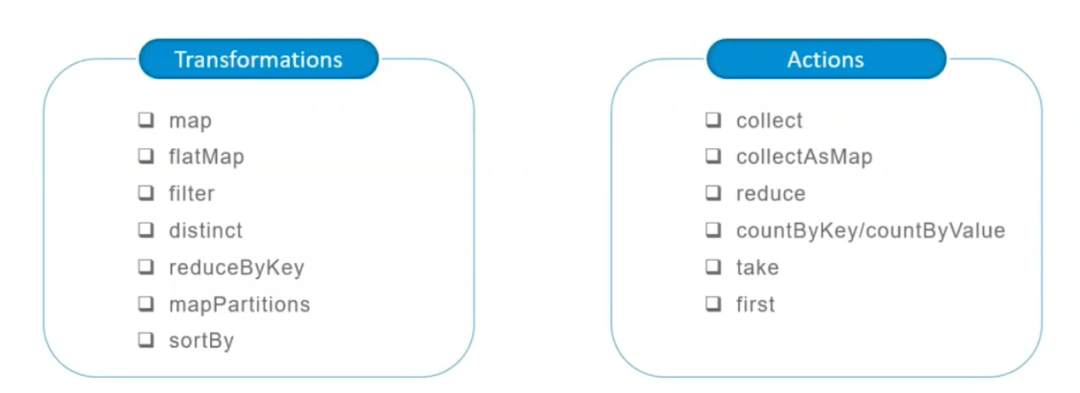import os
import pyspark
from pyspark import SparkContext, SparkConf

conf = SparkConf().setAppName("test_SamShare").setMaster("local")
sc = SparkContext(conf=conf)

# 使用 parallelize方法直接实例化一个RDD
rdd = sc.parallelize(range(1,11),4) # 这里的 4 指的是分区数量
rdd.take(100)
# [1, 2, 3, 4, 5, 6, 7, 8, 9, 10]

"""
----------------------------------------------
Transform算子解析
----------------------------------------------
"""
# 以下的操作由于是Transform操作，因为我们需要在最后加上一个collect算子用来触发计算。
# 1. map: 和python差不多，map转换就是对每一个元素进行一个映射
rdd = sc.parallelize(range(1, 11), 4)
rdd_map = rdd.map(lambda x: x*2)
print("原始数据：", rdd.collect())
print("扩大2倍：", rdd_map.collect())
# 原始数据： [1, 2, 3, 4, 5, 6, 7, 8, 9, 10]
# 扩大2倍： [2, 4, 6, 8, 10, 12, 14, 16, 18, 20]

# 2. flatMap: 这个相比于map多一个flat（压平）操作，顾名思义就是要把高维的数组变成一维
rdd2 = sc.parallelize(["hello SamShare", "hello PySpark"])
print("原始数据：", rdd2.collect())
print("直接split之后的map结果：", rdd2.map(lambda x: x.split(" ")).collect())
print("直接split之后的flatMap结果：", rdd2.flatMap(lambda x: x.split(" ")).collect())
# 直接split之后的map结果： [['hello', 'SamShare'], ['hello', 'PySpark']]
# 直接split之后的flatMap结果： ['hello', 'SamShare', 'hello', 'PySpark']

# 3. filter: 过滤数据
rdd = sc.parallelize(range(1, 11), 4)
print("原始数据：", rdd.collect())
print("过滤奇数：", rdd.filter(lambda x: x % 2 == 0).collect())
# 原始数据： [1, 2, 3, 4, 5, 6, 7, 8, 9, 10]
# 过滤奇数： [2, 4, 6, 8, 10]

# 4. distinct: 去重元素
rdd = sc.parallelize([2, 2, 4, 8, 8, 8, 8, 16, 32, 32])
print("原始数据：", rdd.collect())
print("去重数据：", rdd.distinct().collect())
# 原始数据： [2, 2, 4, 8, 8, 8, 8, 16, 32, 32]
# 去重数据： [4, 8, 16, 32, 2]

# 5. reduceByKey: 根据key来映射数据
rdd = sc.parallelize([("a", 1), ("b", 1), ("a", 1)])
print("原始数据：", rdd.collect())
# 原始数据： [('a', 1), ('b', 1), ('a', 1)]
# 原始数据： [('b', 1), ('a', 2)]

# 6. mapPartitions: 根据分区内的数据进行映射操作
rdd = sc.parallelize([1, 2, 3, 4], 2)
def f(iterator):
yield sum(iterator)
print(rdd.collect())
print(rdd.mapPartitions(f).collect())
# [1, 2, 3, 4]
# [3, 7]

# 7. sortBy: 根据规则进行排序
tmp = [('a', 1), ('b', 2), ('1', 3), ('d', 4), ('2', 5)]
print(sc.parallelize(tmp).sortBy(lambda x: x).collect())
print(sc.parallelize(tmp).sortBy(lambda x: x).collect())
# [('1', 3), ('2', 5), ('a', 1), ('b', 2), ('d', 4)]
# [('a', 1), ('b', 2), ('1', 3), ('d', 4), ('2', 5)]

# 8. subtract: 数据集相减, Return each value in self that is not contained in other.
x = sc.parallelize([("a", 1), ("b", 4), ("b", 5), ("a", 3)])
y = sc.parallelize([("a", 3), ("c", None)])
print(sorted(x.subtract(y).collect()))
# [('a', 1), ('b', 4), ('b', 5)]

# 9. union: 合并两个RDD
rdd = sc.parallelize([1, 1, 2, 3])
print(rdd.union(rdd).collect())
# [1, 1, 2, 3, 1, 1, 2, 3]

# 10. interp: 取两个RDD的交集，同时有去重的功效
rdd1 = sc.parallelize([1, 10, 2, 3, 4, 5, 2, 3])
rdd2 = sc.parallelize([1, 6, 2, 3, 7, 8])
print(rdd1.interp(rdd2).collect())
# [1, 2, 3]

# 11. cartesian: 生成笛卡尔积
rdd = sc.parallelize([1, 2])
print(sorted(rdd.cartesian(rdd).collect()))
# [(1, 1), (1, 2), (2, 1), (2, 2)]

# 12. zip: 拉链合并，需要两个RDD具有相同的长度以及分区数量
x = sc.parallelize(range(0, 5))
y = sc.parallelize(range(1000, 1005))
print(x.collect())
print(y.collect())
print(x.zip(y).collect())
# [0, 1, 2, 3, 4]
# [1000, 1001, 1002, 1003, 1004]
# [(0, 1000), (1, 1001), (2, 1002), (3, 1003), (4, 1004)]

# 13. zipWithIndex: 将RDD和一个从0开始的递增序列按照拉链方式连接。
rdd_name = sc.parallelize(["LiLei", "Hanmeimei", "Lily", "Lucy", "Ann", "Dachui", "RuHua"])
rdd_index = rdd_name.zipWithIndex()
print(rdd_index.collect())
# [('LiLei', 0), ('Hanmeimei', 1), ('Lily', 2), ('Lucy', 3), ('Ann', 4), ('Dachui', 5), ('RuHua', 6)]

# 14. groupByKey: 按照key来聚合数据
rdd = sc.parallelize([("a", 1), ("b", 1), ("a", 1)])
print(rdd.collect())
print(sorted(rdd.groupByKey().mapValues(len).collect()))
print(sorted(rdd.groupByKey().mapValues(list).collect()))
# [('a', 1), ('b', 1), ('a', 1)]
# [('a', 2), ('b', 1)]
# [('a', [1, 1]), ('b', )]

# 15. sortByKey:
tmp = [('a', 1), ('b', 2), ('1', 3), ('d', 4), ('2', 5)]
print(sc.parallelize(tmp).sortByKey(True, 1).collect())
# [('1', 3), ('2', 5), ('a', 1), ('b', 2), ('d', 4)]

# 16. join:
x = sc.parallelize([("a", 1), ("b", 4)])
y = sc.parallelize([("a", 2), ("a", 3)])
print(sorted(x.join(y).collect()))
# [('a', (1, 2)), ('a', (1, 3))]

# 17. leftOuterJoin/rightOuterJoin
x = sc.parallelize([("a", 1), ("b", 4)])
y = sc.parallelize([("a", 2)])
print(sorted(x.leftOuterJoin(y).collect()))
# [('a', (1, 2)), ('b', (4, None))]

"""
----------------------------------------------
Action算子解析
----------------------------------------------
"""
# 1. collect: 指的是把数据都汇集到driver端，便于后续的操作
rdd = sc.parallelize(range(0, 5))
rdd_collect = rdd.collect()
print(rdd_collect)
# [0, 1, 2, 3, 4]

# 2. first: 取第一个元素
sc.parallelize([2, 3, 4]).first()
# 2

# 3. collectAsMap: 转换为dict，使用这个要注意了，不要对大数据用，不然全部载入到driver端会爆内存
m = sc.parallelize([(1, 2), (3, 4)]).collectAsMap()
m
# {1: 2, 3: 4}

# 4. reduce: 逐步对两个元素进行操作
rdd = sc.parallelize(range(10),5)
print(rdd.reduce(lambda x,y:x+y))
# 45

# 5. countByKey/countByValue:
rdd = sc.parallelize([("a", 1), ("b", 1), ("a", 1)])
print(sorted(rdd.countByKey().items()))
print(sorted(rdd.countByValue().items()))
# [('a', 2), ('b', 1)]
# [(('a', 1), 2), (('b', 1), 1)]

# 6. take: 相当于取几个数据到driver端
rdd = sc.parallelize([("a", 1), ("b", 1), ("a", 1)])
print(rdd.take(5))
# [('a', 1), ('b', 1), ('a', 1)]

# 7. saveAsTextFile: 保存rdd成text文件到本地
text_file = "./data/rdd.txt"
rdd = sc.parallelize(range(5))
rdd.saveAsTextFile(text_file)

# 8. takeSample: 随机取数
rdd = sc.textFile("./test/data/hello_samshare.txt", 4)  # 这里的 4 指的是分区数量
rdd_sample = rdd.takeSample(True, 2, 0)  # withReplacement 参数1：代表是否是有放回抽样
rdd_sample

# 9. foreach: 对每一个元素执行某种操作，不生成新的RDD
rdd = sc.parallelize(range(10), 5)
accum = sc.accumulator(0)
print(accum.value)
# 45

5.数据读取与保存
5.1 文本文件
读取文本文件，保存文件
data=sc.textFile("file://home/README.md")
data.saveAsTextFile(outputFile)

5.2 JSON文件
import json
data.filter(lambda x:x["lovesPandas"]).map(lambda x:json.dumps(x)).saveAsTextFile(outputFile)

5.3 逗号分隔值与制表符分隔值
import csv
import StringIO
input=StringIO.stringIO(line)



展开全文大数据
• 在这个课程中，我们会学习如何编写并且调试Python Spark（pySpark）程序。为了满足大家的需求，我们的软件开发环境是使用Virtual Machine（VM虚拟机）。本文将手把手教你安装该环境。python spark
• Python调用pyspark 安装寻找spark的库findspark pip install findspark word count示例 import findspark findspark.init() from pyspark import SparkConf, SparkContext # 创建SparkConf和SparkContext conf = ...
• ## pyspark使用教程（一）

万次阅读 多人点赞 2019-03-18 20:18:00
使用Pyspark教程，参考《Spark快速大数据分析》 1.Spark背景 Apache Spark是专为大规模数据处理而设计的快速通用的计算引擎。Spark是UC Berkeley AMP lab (加州大学伯克利分校的AMP实验室)所开源的类Hadoop ...
• ## pyspark入门教程

千次阅读 多人点赞 2020-07-20 20:18:21
一、windows下配置pyspark环境 1.1 jdk下载安装 1.2 Scala下载安装 1.3 spark下载安装 1.4 Hadoop下载安装 1.5 pyspark下载安装 1.6 anaconda下载安装 1.7 测试环境是否搭建成功 二、pyspark原理简介 三、...python spark 数据分析 数据挖掘
• ## pyspark基础教程

千次阅读 2018-03-03 14:04:03
pyspark基础教程 下面一段代码是简单教程,对与如何向spark 集群提交代码任务,无论文档和博客都有很多说法,其实很简单,只要在脚本中setMaster(“spark://192.168.10.182:7077”), spark://192.168.10.182:7077是...
• ## pyspark-教程

千次阅读 2017-10-16 11:07:30
参考： 1、https://github.com/mahmoudparsian/pyspark-tutorial ...Download, Install Spark and Run PySpark Basics of PySpark PySpark Examples and Tutorials DNA Base CountingClassic Word CountFindspark
• 以一个处理结构化数据的入门程序，带大家进入pyspark的大门。本例子以深圳股市的股息率分析为例，讲解spark的输入、分析计算和输出。...